• 1.单个ppp元正态总体协方差阵的检验 具体步骤： 作统计假设(1)：H0:∑=Ip,H1:∑≠IpH0:∑=Ip,H1:∑≠IpH_0:\sum =I_p,H_1:\sum \neq I_p 统计量：λ=supθ∈Θ0L(μ,Ip)supθ∈ΘL(μ,∑)=(en)np/2|S|n/2exp[−12...
1.单个p$p$$p$元正态总体协方差阵的检验

具体步骤：

作统计假设(1)：H0:∑=Ip,H1:∑≠Ip${H}_{0}:\sum ={I}_{p},{H}_{1}:\sum \ne {I}_{p}$$H_0:\sum =I_p,H_1:\sum \neq I_p$
统计量：λ=supθ∈Θ0L(μ,Ip)supθ∈ΘL(μ,∑)=(en)np/2|S|n/2exp[−12tr(S)]$\lambda =\frac{\underset{\theta \in {\mathrm{\Theta }}_{0}}{sup}L\left(\mu ,{I}_{p}\right)}{\underset{\theta \in \mathrm{\Theta }}{sup}L\left(\mu ,\sum \right)}=\left(\frac{e}{n}{\right)}^{np/2}{|S|}^{n/2}exp\left[-\frac{1}{2}tr\left(S\right)\right]$\lambda=\frac{\underset{\theta\in \Theta _0}{sup}{L(\mu,I_p)}}{\underset{\theta\in \Theta }{sup}{L(\mu,\sum)}}=(\frac{e}{n})^{np/2}\left| S\right|^{n/2}exp\left[-\frac{1}{2}tr(S) \right ]Λ=−2lnλ∼χ2(p(p+1)2)$\mathrm{\Lambda }=-2ln\lambda \sim {\chi }^{2}\left(\frac{p\left(p+1\right)}{2}\right)$\Lambda=-2ln\lambda \sim \chi^2(\frac{p(p+1)}{2})其中supθ∈Θ0L(μ,Ip)=L(X¯,Ip)=(2π)−np/2exp[−12tr(S)]$\underset{\theta \in {\mathrm{\Theta }}_{0}}{sup}L\left(\mu ,{I}_{p}\right)=L\left(\overline{X},{I}_{p}\right)=\left(2\pi {\right)}^{-np/2}exp\left[-\frac{1}{2}tr\left(S\right)\right]$\underset{\theta\in \Theta _0}{sup}{L(\mu,I_p)}=L(\bar{X},I_p)=(2\pi)^{-np/2}exp\left[-\frac{1}{2}tr(S) \right ]supθ∈ΘL(μ,∑)=L(X¯,Sn)=(2π)−np/2∣∣∣1nS∣∣∣−n/2exp[−12pn]$\underset{\theta \in \mathrm{\Theta }}{sup}L\left(\mu ,\sum \right)=L\left(\overline{X},\frac{S}{n}\right)=\left(2\pi {\right)}^{-np/2}{|\frac{1}{n}S|}^{-n/2}exp\left[-\frac{1}{2}pn\right]$\underset{\theta\in \Theta }{sup}{L(\mu,\sum)}=L(\bar{X},\frac{S}{n})=(2\pi)^{-np/2}\left| \frac{1}{n}S\right|^{-n/2}exp\left[-\frac{1}{2}pn \right ]
作统计假设(2)：H0:∑=∑0,H1:∑≠∑0${H}_{0}:\sum =\sum _{0},{H}_{1}:\sum \ne \sum _{0}$$H_0:\sum =\sum_0,H_1:\sum \neq \sum_0$
统计量：做变换转换为上面的假设：Yi=CXi,C${Y}_{i}=C{X}_{i},C$$Y_i=CX_i,C$为非奇异矩阵λ=(en)np/2|SY|n/2exp[−12tr(SY)]$\lambda =\left(\frac{e}{n}{\right)}^{np/2}{|{S}_{Y}|}^{n/2}exp\left[-\frac{1}{2}tr\left({S}_{Y}\right)\right]$\lambda=(\frac{e}{n})^{np/2}\left| S_Y\right|^{n/2}exp\left[-\frac{1}{2}tr(S_Y) \right ]其中SY=CS′C${S}_{Y}=C{S}^{\prime }C$$S_Y=CS'C$
作统计假设(3)：（球性检验）H0:∑=σ2∑0${H}_{0}:\sum ={\sigma }^{2}\sum _{0}$$H_0:\sum =\sigma^2\sum_0$(σ2未知${\sigma }^{2}未知$$\sigma^2未知$)
统计量：
σ2${\sigma }^{2}$$\sigma^2$的极大似然估计为1nptr(∑−10S)$\frac{1}{np}tr\left(\sum _{0}^{-1}S\right)$$\frac{1}{np}tr(\sum_0^{-1}S)$
λ=∣∣∑−10S∣∣n/2[tr(∑−10S)/p]np/2$\lambda =\frac{{|\sum _{0}^{-1}S|}^{n/2}}{{\left[tr\left(\sum _{0}^{-1}S\right)/p\right]}^{np/2}}$\lambda=\frac{{\left| \sum_0^{-1}S \right|}^{n/2}}{{\left[tr(\sum_0^{-1}S)/p \right]}^{np/2}}W=(λ)2/n=pp∣∣∑−10S∣∣[tr(∑−10S)]p$W=\left(\lambda {\right)}^{2/n}=\frac{{p}^{p}|\sum _{0}^{-1}S|}{{\left[tr\left(\sum _{0}^{-1}S\right)\right]}^{p}}$W=(\lambda)^{2/n}=\frac{p^p\left| \sum_0^{-1}S \right|}{{\left[tr(\sum_0^{-1}S) \right]}^p}−((n−1)−2p2+p+26p)lnW∼χ2(p(p+1)2−1)$-\left(\left(n-1\right)-\frac{2{p}^{2}+p+2}{6p}\right)lnW\sim {\chi }^{2}\left(\frac{p\left(p+1\right)}{2}-1\right)$-((n-1)-\frac{2p^2+p+2}{6p})lnW\sim \chi^2(\frac{p(p+1)}{2}-1)

*R实现：

#### variance testing #########
var.test=function(data, Sigma0)
###############################################################
## H0: Sigma=Sigma0
## this is aymptotically a Chisq testing
##############  Input  ########################################
## data  = design matrix with the ith sample in the ith line
## Sigma0= Simga0 for null hypothesis
############## Output  ########################################
## p.value     = p value
###############################################################
{
n=nrow(data)
p=ncol(data)

A=(n-1)*var(data)
S=A%*%solve(Sigma0)

lambda=exp(sum(diag((-1)*S/2)))*(det(S))^(n/2)*(exp(1)/n)^(n*p/2)
T5=-2*log(lambda)

p.value=1-pchisq(T5, p*(p+1)/2)
return(p.value)
}

2.两个p$p$$p$ 元正态总体协方差阵相等的检验

具体步骤：

作统计假设：H0:∑1=∑2,H1:∑1≠∑2${H}_{0}:\sum _{1}=\sum _{2},{H}_{1}:\sum _{1}\ne \sum _{2}$$H_0:\sum_1=\sum_2,H_1:\sum_1\neq \sum_2$
统计量：（式子太长有时间补上）

*R实现：

#### k independent normal distribution  #########
multi.var.test=function(data, k)
###################################################################
## H0: Sigma1=Sigma2=...=Sigmak
## this is asymptotically a Chisq testing
##############  Input  ############################################
## data  = design matrix with a group index ind
############## Output  ############################################
## p.value     = p value
###################################################################
{
ind=data$ind n=nrow(data) p=ncol(data)-1 data=data[ ,1:p] A=0 for (i in 1:k) { datai=data[ind==i, ] ni=nrow(datai) A=A+(ni-1)*var(datai) } det.A=0 for (i in 1:k) { datai=data[ind==i, ] ni=nrow(datai) det.A=det.A+(ni-1)*log(det(var(datai))) } M=(n-k)*log(det(A/(n-k)))-det.A d=(2*p^2+3*p-1)*(k+1)/(6*(p+1)*(n-k)) f=p*(p+1)*(k-1)/2 T6=(1-d)*M p.value=1-pchisq(T6, f) return(p.value=p.value) }  3.独立性检验 （二个总体） X∼Np(μ,∑),X={X1X2},X1:q,X2:p−q$X\sim {N}_{p}\left(\mu ,\sum \right),X=\left\{\begin{array}{c}{X}_{1}\\ {X}_{2}\end{array}\right\},{X}_{1}:q,{X}_{2}:p-q$$X\sim N_p(\mu,\sum),X=\begin{Bmatrix}X_1\\ X_2\end{Bmatrix} ,X_1:q,X_2:p-q$ 具体步骤： 作统计假设：H0:∑12=0${H}_{0}:\sum _{12}=0$$H_0:\sum_{12}=0$ 统计量：记∑0={∑1100∑22}$记\sum _{0}=\left\{\begin{array}{cc}\sum _{11}& 0\\ 0& \sum _{22}\end{array}\right\}$记\sum_0=\begin{Bmatrix} \sum_{11} & 0 \\ 0& \sum_{22} \end{Bmatrix}S=∑i=1n(Xi−X¯)(Xi−X¯)′={S11S21S12S22}$S=\sum _{i=1}^{n}\left({X}_{i}-\overline{X}\right)\left({X}_{i}-\overline{X}{\right)}^{\prime }=\left\{\begin{array}{cc}{S}_{11}& {S}_{12}\\ {S}_{21}& {S}_{22}\end{array}\right\}$S=\sum_{i=1}^{n}(X_i-\bar{X})(X_i-\bar{X})'=\begin{Bmatrix} S_{11} & S_{12} \\ S_{21}& S_{22} \end{Bmatrix}λ2/n=|S||S11||S22|∼Λ(p−q)${\lambda }^{2/n}=\frac{|S|}{|{S}_{11}||{S}_{22}|}\sim \mathrm{\Lambda }\left(p-q\right)$\lambda^{2/n}=\frac{\left| S \right|}{\left| S_{11}\right|\left| S_{22}\right|}\sim \Lambda(p-q) 对K个总体：V=|S|∏ki=1|Sii|=∏i=1k−1vi,vi∼Λ(mi,n−∑j=1imj−1,∑j=1imj)$V=\frac{|S|}{\prod _{i=1}^{k}|{S}_{ii}|}=\prod _{i=1}^{k-1}{v}_{i},{v}_{i}\sim \mathrm{\Lambda }\left({m}_{i},n-\sum _{j=1}^{i}{m}_{j}-1,\sum _{j=1}^{i}{m}_{j}\right)$V=\frac{\left| S \right|}{\prod_{i=1}^{k}\left| S_{ii} \right|}=\prod_{i=1}^{k-1}v_i,v_i\sim \Lambda(m_i,n-\sum_{j=1}^{i}m_j-1,\sum_{j=1}^{i}m_j)−blnV∼χ2(f)$-blnV\sim {\chi }^{2}\left(f\right)$-blnV\sim \chi^2(f)其中b=n−32−p3−∑k1p3i3(p2−∑k1p2i)$b=n-\frac{3}{2}-\frac{{p}^{3}-\sum _{1}^{k}{p}_{i}^{3}}{3\left({p}^{2}-\sum _{1}^{k}{p}_{i}^{2}\right)}$b=n-\frac{3}{2}-\frac{p^3-\sum_{1}^{k}p_i^3}{3(p^2-\sum_{1}^{k}p_i^2)}f=12[p(p+1)−∑1kpi(pi+1)]$f=\frac{1}{2}\left[p\left(p+1\right)-\sum _{1}^{k}{p}_{i}\left({p}_{i}+1\right)\right]$f=\frac{1}{2}[p(p+1)-\sum_{1}^{k}p_i(p_i+1)] *R实现： ######### testing for independent ######### multi.ind.test=function(data, k) ################################################################### ## H0: sigma(ij)=0,i!=j ## this is asymptotically a Chisq testing ############## Input ############################################ ## data = design matrix with a group index ind ############## Output ############################################ ## p.value = p value ################################################################### { ind=data$ind #不同总体分组#

n=nrow(data)-1 #因为最后一行为ind#
p=ncol(data)
data=data[1:n , ]
X.bar=apply(data, 2, mean)
S=(n-1)*var(data)

det.B=1
P1=0
P2=0
P3=0
for (i in 1:k)
{
datai=data[ ,ind==i]
p_i=ncol(datai)
P1=P1+p_i**3
P2=P2+p_i**2
P3=P3+p_i*(p_i+1)
Si=(n-1)*var(datai)
det.B=det.Si*det.B
}

V=det.S/det.B
b=n-3/2-(p^3-P1)/(3*p^2-3*P2)
f=0.5*(p(p+1)-P3)

T6=(-b)*log(V)

p.value=1-pchisq(T6, f)
return(p.value=p.value)
}

展开全文• ## 透彻理解多元正态分布

千次阅读 多人点赞 2020-03-14 14:50:52
本篇内容主要是对于基本书籍教材多元正态分布相关章节所写的学习笔记，结合自己的理解尽可能表述得通俗易懂，主要思路内容取自《程序员的数学之概率统计》。 前言 多元正态分布就是含有多个变量的正态分布，为什么...
文章目录前言多元标准正态分布多元一般正态分布缩放与位移相同尺度缩放与位移不同尺度旋转变换多元正态分布的概率密度函数多元正态分布的性质
本篇内容主要是对于基本书籍教材多元正态分布相关章节所写的学习笔记，结合自己的理解尽可能表述得通俗易懂，主要思路内容取自《程序员的数学之概率统计》。
前言
多元正态分布就是含有多个变量的正态分布，为什么关于多元正态分布要专门写一篇学习笔记？因为其具有重要意义，在理论研究或者实际应用中，我们常会首先考虑多元正态分布是否适用，如果不符，再考虑其他类型的分布。基于下面两个特征，多元正态分布应用十分广泛：

多元正态分布的表达式易于处理，且理论推导的结果较为简洁
现实生活中很多问题都能通过多元正态分布解释或近似

多元正态分布的数学形式复杂，但大多情况下都可以通过椭圆或椭圆体表述，这就是学习时需要具备的几何理解。
多元标准正态分布
定义：如果列向量$Z=(Z_1,\dots,Z_n)^T$由n个遵从标准正态分布的随机变量$Z_1,\dots,Z_n$组成，那么称Z遵从n元标准正态分布。二元标准正态分布（均值0方差1）的概率密度及概率密度函数如下图所示：当$Z_1,\dots,Z_n$互相独立时，概率密度函数如下：
$f_{Z}(z)=g\left(z_{1}\right) g\left(z_{2}\right) \cdots g\left(z_{n}\right)$
这里的g是标准正态分布的概率密度函数，具体形式如下：
$f_{Z}(z)=c \exp \left(-\frac{z_{1}^{2}}{2}\right) \cdot c \exp \left(-\frac{z_{2}^{2}}{2}\right) \cdots c \exp \left(-\frac{z_{n}^{2}}{2}\right)$
这里的c是根据总概率为1这一条件所求得的常量。整理上式可得到如下表达式：
$f_{Z}(z)=d \exp \left(-\frac{1}{2}\|z\|^{2}\right)$
这就是n元标准正态分布的概率密度函数。d仍是由总概率为1的条件求得的常量。（$c\int_{-\infty}^{\infty} \exp \left(-z^{2} / 2\right) d z=1$，$c=1/\sqrt{2\pi}$；$d=c^n$这就是c和d的具体值）
观察n元标准正态分布的概率密度函数，向量z的长度为：
$\|z\|=\sqrt{z_{1}^{2}+z_{2}^{2}+\cdots+z_{n}^{2}}=\sqrt{z^{T} z}$
由此可发现，概率密度函数$f_Z(z)$的等高线是一个圆或者等值面试一个球面或超球面（这里超球面或者等值线就是所有函数值相同的点连接得到的图形）。
为什么是圆或者球面超球面可以这么理解：$f_Z(z)$的表达式中的自变量其实就是$||z||$，也就是说，即使不知道向量z具体值，只要确定其长度，就可以计算得到$f_Z(z)$。所以只要向量长度相同，函数值就相同，也就是以原点为中心的圆周上任意位置的函数值都相同，这就是球面超球面圆的定义。
确定Z的概率密度函数之后，再来求期望值向量与协方差矩阵。以n=3的情况为例：
$\begin{array}{l} \mathrm{E}[\boldsymbol{Z}]=\left(\begin{array}{c} \mathrm{E}\left[Z_{1}\right] \\ \mathrm{E}\left[Z_{2}\right] \\ \mathrm{E}\left[Z_{3}\right] \end{array}\right)=\left(\begin{array}{l} 0 \\ 0 \\ 0 \end{array}\right)=o \\ \mathrm{V}[\boldsymbol{Z}]=\left(\begin{array}{ccc} \mathrm{V}\left[Z_{1}\right] & \operatorname{Cov}\left[Z_{1}, Z_{2}\right] & \operatorname{Cov}\left[Z_{1}, Z_{3}\right] \\ \operatorname{Cov}\left[Z_{2}, Z_{1}\right] & \mathrm{V}\left[Z_{2}\right] & \operatorname{Cov}\left[Z_{2}, Z_{3}\right] \\ \operatorname{Cov}\left[Z_{3}, Z_{1}\right] & \operatorname{Cov}\left[Z_{3}, Z_{2}\right] & \mathrm{V}\left[Z_{3}\right] \end{array}\right)=\left(\begin{array}{lll} 1 & 0 & 0 \\ 0 & 1 & 0 \\ 0 & 0 & 1 \end{array}\right) \end{array}$
推广到n元的情况也是一样，期望值是n元零向量$o$，协方差矩阵是n元单位矩阵$I$。综上，即可通过$Z \sim N(o,I)$表示Z遵从n元标准正态分布。有以下性质：

各元素标准差均为1
不仅坐标轴方向，任意方向标准差都为1

这里由上面已证明的等高线是圆，可从第一条推出第二条。
多元一般正态分布
一般正态分布可由标准正态分布通过平移或缩放得到，同理，多元一般正态分布也可以由多元标准正态分布通过变换得到。在讨论之前先看一下如何通过转换随机变量X来获得需要的期望和方差。假如有两个随机变量Y和Z，他们与X的关系满足$Y=X+c$，$Z=cX$。那么他们的方差和均值变化的结果如下，先看Y的情况：
$E[Y]=E[X+c]=E[X]+c, V[Y]=V[X+c]=V[X]$
再看Z的情况：
$E[Z]=E[cX]=cE[X], V[Z]=V[cX]=c^2V[X]$
根据这些性质，就可以通过转换随机变量X来获得需要的期望值与方差了。例如X的期望为$\mu$方差为$\sigma^2$，此时只要令$W=\frac{X-\mu}{\sigma}$即可得到期望为0，方差为1的分布了。这个令期望为0方差为1的转换处理过程就叫做标准化（或者归一化）。
缩放与位移相同尺度
设$X=\sigma Z+\mu$，其中$\sigma$是一个正的常量，$\mu$是一个n元的常向量。此时，X的期望值与方差如下：
\begin{aligned} &\mathrm{E}[\boldsymbol{X}]=\sigma \mathrm{E}[\boldsymbol{Z}]+\boldsymbol{\mu}=\boldsymbol{\mu}\\ &\mathrm{V}[\boldsymbol{X}]=\sigma^{2} \mathrm{V}[\boldsymbol{Z}]=\sigma^{2} I=\left(\begin{array}{ccc} \sigma^{2} & & \\ & \ddots & \\ & & \sigma^{2} \end{array}\right) \end{aligned}
X服从的分布就称为“期望值为$\mu$且协方差矩阵为$\sigma^2 I$的n元正态分布”，记作$X \sim N(\mu, \sigma^2 I)$。二元情况下其概率密度与概率密度函数如下图所示：上面右图的体积为1，基准圆圆心变为$\mu$，半径变为$\sigma$。
缩放与位移不同尺度
上一部分的缩放中，所有方向的缩放程度是相等的。如果不同坐标轴缩放倍率不同，那就会得到一个椭圆状分布。基准圆也会变为椭圆。还是以列向量$Z=(Z_1,\dots,Z_n)^T$为例，如果对Z的各个元素分别缩放不同倍，得到$\boldsymbol{X} =\left(\sigma_{1} Z_{1}, \cdots, \sigma_{n} Z_{n}\right)^{T}$，这一变换的矩阵形式如下：
$\boldsymbol{X}=D \boldsymbol{Z}, \quad D \equiv\left(\begin{array}{ccc} \sigma_{1} & & \\ & \ddots & \\ & & \sigma_{n} \end{array}\right)$
此时X的协方差矩阵是如下对角阵：
$\mathrm{V}[\boldsymbol{X}]=D^{2}=\left(\begin{array}{ccc} \sigma_{1}^{2} & & \\ & \ddots & \\ & & \sigma_{n}^{2} \end{array}\right)$
协方差矩阵的求解推导过程如下（如果对下面推导过程不明白，可参考之前博文协方差与协方差矩阵内容）：
$\mathrm{V}[\boldsymbol{X}]=\mathrm{V}[D \boldsymbol{Z}]=D \mathrm{V}[\boldsymbol{Z}] D^{T}=D I D^{T}=D^{2}$
如果再加上常向量$\mu$来使其在各个坐标轴方向上平移不同的距离，那么期望值向量会增加$\mu$，而协方差矩阵不变。此时的分布就是一般的多元正态分布的形式，记作$N(\mu,D^2)$。其概率密度与概率密度函数如下图所示：旋转变换
旋转已有的分布后得到的将是更加一般的多元正态分布，通常我们使用正交矩阵的乘法运算来表示旋转变换。如果X是以原点为中心的多元正态分布，有正交矩阵Q使得$Y=QX$，那么：
$\begin{array}{l} \mathrm{E}[Y]=Q \mathrm{E}[X]=o \\ \mathrm{V}[Y]=Q \mathrm{V}[X] Q^{T}=Q D^{2} Q^{T} \end{array}$
由此就得到了一个协方差矩阵不是对角阵的一般多元正态分布。反之，如果希望某个分布属于多元正态分布（如果希望得到的多元正态分布具有符合要求的协方差矩阵V），那么就令其协方差矩阵符合$V=QD^2Q^T$这个条件，其中Q为正交阵，D为对角阵。
注意，这个条件和$Q^TVQ=D^2$是等价的。又因为协方差矩阵V是一个对称阵，所以这个条件可以描述为：对于给定的对称阵V，找到一个合适的正交矩阵Q，使得$Q^TVQ$是一个对角阵。这就是通过对称矩阵和正交矩阵实现矩阵对角化的方法。依据的是该定理：如果一个矩阵H是对称矩阵，那么必然存在正交矩阵Q，使得$Q^THQ$为对角阵。这个对角阵的每一个对角元素都是特征值，每个特征值对应的Q中的向量都是特征向量。
解出对角阵后只需使$D^{2}=\operatorname{diag}\left(\lambda_{1}, \cdots, \lambda_{n}\right)$即可解得D为：
$D = \left(\begin{array}{ccc} \sqrt{\lambda_{1}} & & \\ & \ddots & \\ & & \sqrt{\lambda_{n}} \end{array}\right)$
由此将D与Q代入上面的V的表达式中，即可得到多元正态分布$N(o, V)$。再加上常向量$\mu$即可实现平移，得到最一般的多元正态分布$N(\mu,V)$。其概率密度与概率密度函数示意图如下：多元正态分布的概率密度函数
为讨论多元正态分布的各种方便的性质，首先需要知道多元正态分布的概率密度函数。对于n元标准正态分布上面已经推导过其概率密度函数如下：
$f_{Z}(z)=\frac{1}{\sqrt{2 \pi}^{n}} \exp \left(-\frac{1}{2}\|z\|^{2}\right)$
如何对Z进行变换，从而得到一个协方差矩阵为$V=QD^2Q^T$的一般n元正态分布呢？
先令
$Y=QDZ$
这里的Q是正交阵，D是对角线元素全部都为正的对角阵。由于Q和D都是正规矩阵，所以它们的乘积QD也是正规矩阵。
这里再补充一个知识点，即多变量乘以一个正规矩阵变换之后的新变量的概率密度函数和原概率密度函数的关系。该知识点可进行如下描述。
对于$Z_1=g_1(X_1,\dots,X_n), \dots, Z_n=g_n(X_1,\dots,X_n)$的概率密度函数f有以下结论：
$f_{Z_{1}, \cdots, Z_{n}}\left(z_{1}, \cdots, z_{n}\right)=\left|\frac{\partial\left(x_{1}, \cdots, x_{n}\right)}{\partial\left(z_{1}, \cdots, z_{n}\right)}\right| f_{X_{1}, \cdots, X_{n}}\left(x_{1}, \cdots, x_{n}\right)$
其中
$\frac{\partial\left(x_{1}, \cdots, x_{n}\right)}{\partial\left(z_{1}, \cdots, z_{n}\right)} \equiv \operatorname{det}\left(\begin{array}{ccc} \frac{\partial x_{1}}{\partial z_{1}} & \cdots & \frac{\partial x_{1}}{\partial z_{n}} \\ \vdots & & \vdots \\ \frac{\partial x_{n}}{\partial z_{1}} & \cdots & \frac{\partial x_{n}}{\partial z_{n}} \end{array}\right)=\frac{1}{\partial\left(z_{1}, \cdots, z_{n}\right) / \partial\left(x_{1}, \cdots, x_{n}\right)}$
这个知识点讲述了这么一个情况：以二维为例，x和y组成了表示概率密度的平面网格点，而z轴则是概率密度函数值，其和xy平面围成的体积必须为1。当把xy进行线性变换之后，网格的大小也会有倍数的扩大，这个面积的变化就叫做面积扩大率，其数值为$|detA|$。既然面积扩大了，那么为了维持体积不变，概率密度函数值就需要缩小相应的倍数。所以，多元标准正态分布的随机变量乘以$A=QD$之后，概率密度函数就会改变为：
$f_{\boldsymbol{Y}}(\boldsymbol{y})=\frac{1}{|\operatorname{det} A|} f_{\boldsymbol{Z}}\left(A^{-1} \boldsymbol{y}\right)=\frac{1}{|\operatorname{det} A|} \cdot \frac{1}{\sqrt{2 \pi}^{n}} \exp \left(-\frac{1}{2}\left\|A^{-1} \boldsymbol{y}\right\|^{2}\right)$
这还不够，这个概率密度函数中体现不出方差的概念。对上面等式右边进行整理，尝试用协方差矩阵V来表示。首先根据下面关系（如果对下面推导过程不明白，可参考之前博文协方差与协方差矩阵内容）：
$V=\mathrm{V}[A Z]=A \mathrm{V}[Z] A^{T}=A I A^{T}=A A^{T}$
可得到如下结论
$\operatorname{det} V=\operatorname{det}\left(A A^{T}\right)=(\operatorname{det} A)\left(\operatorname{det} A^{T}\right)=(\operatorname{det} A)^{2}$
又由于$V^{-1}=\left(A A^{T}\right)^{-1}=\left(A^{T}\right)^{-1} A^{-1}=\left(A^{-1}\right)^{T} A^{-1}$，可得到如下结果
$\left\|A^{-1} \boldsymbol{y}\right\|^{2}=\left(A^{-1} \boldsymbol{y}\right)^{T}\left(A^{-1} \boldsymbol{y}\right)=\boldsymbol{y}^{T}\left(A^{-1}\right)^{T} A^{-1} \boldsymbol{y}=\boldsymbol{y}^{T} V^{-1} \boldsymbol{y}$
综上，最终结果为：
$f_{Y}(y)=\frac{1}{\sqrt{(2 \pi)^{n} \operatorname{det} V}} \exp \left(-\frac{1}{2} y^{T} V^{-1} y\right)$
这就得到了期望值为o的n元正态分布$N(o,V)$的概率密度函数。
这里还有局限性，因为期望值是0，为得到更一般的多元正态分布概率密度函数表达式，令Y位移至$\hat Y=Y+\mu$，即可得到期望值为$\mu$的n元正态分布了，由于只是位移，所以面积和体积都不会发生变化，于是其概率密度函数变化如下
$f_{\tilde{Y}}(\tilde{y})=f_{Y}(\tilde{y}-\mu)=\frac{1}{\sqrt{(2 \pi)^{n} \operatorname{det} V}} \exp \left(-\frac{1}{2}(\tilde{y}-\mu)^{T} V^{-1}(\tilde{y}-\mu)\right)$
综上，最终可得到n元正态分布的概率密度函数为：
$f(\boldsymbol{x})=\frac{1}{\sqrt{(2 \pi)^{n} \operatorname{det} V}} \exp \left(-\frac{1}{2}(\boldsymbol{x}-\boldsymbol{\mu})^{T} V^{-1}(\boldsymbol{x}-\boldsymbol{\mu})\right)$
如果觉得上面公式太复杂，可以抽象为如下形式：
$f(x)=C \exp(X元素的二次式)$
反之，如果概率密度形如上式，则就可以确定X的分布是一种正态分布。这与一元情况同理，对二次式进行配平得方差期望，之后根据体积为1求得常量。
多元正态分布的性质
多元正态分布具有下面三个良好的性质。

可由期望值向量和协方差矩阵确定具体分布
如果各随机变量不相关，则一定独立
多元正态分布经过线性变换之后还是多元正态分布
多元正态分布的条件分布也是多元正态分布
多元正态分布的边缘分布也是多元正态分布

对于第一条性质，显然成立。只要计算期望值向量和协方差矩阵将其代入上一部分求出的概率密度函数公式即可解得概率密度函数。
对于第二条性质，解释之前首先要明确一个概念：
如果随机变量X和Y相互独立，那么其协方差为0，即相关系数为0。
如果随机变量X和Y的协方差为0，即相关系数为0，那么X和Y不一定相互独立。
但是，如果X和Y组成二元正态分布，就可以由协方差等于0直接推得X与Y独立。理由如下。
因为协方差是0，所以协方差矩阵V为对角阵，所以其逆矩阵也是对角阵，两个随机变量方差分别为$\sigma$和$\tau$，那么
\begin{aligned} f_{X}(x) &=\operatorname{\square exp}\left(-\square(x-\mu)^{T} V^{-1}(x-\mu)\right)=\square \exp \left(-\square \frac{(x-\mu)^{2}}{\sigma^{2}}-\square \frac{(y-\nu)^{2}}{\tau^{2}}\right) \\ &=\operatorname{\square exp}\left(-\square \frac{(x-\mu)^{2}}{\sigma^{2}}\right) \exp \left(-\square \frac{(y-\nu)^{2}}{\tau^{2}}\right) \end{aligned}
其中$\square$代表无关紧要的常量，上式可以分解为仅含x的式子和仅含y的式子。这就表明了两个随机变量相互独立，为什么呢？

这里看一下独立性的定义。
独立性有多种表述方式，其中最易于理解的就是“无论是否附加条件，分布都不会发生变化”，那对于随机变量X和Y，这个表述的数学表达形式就是，如果下式始终成立，则称X与Y独立。
$f_{Y|X}(b|a)=f_Y(b)$
这个表达式等价于：
$f_{X,Y}(a,b)=f_X(a)f_Y(b)$

如果随机变量超过两个，该结论依然成立。因为最终$V[X]$是一个对角阵，只要它是对角阵，就可以将概率密度函数分解为仅含单个随机变量的n个正态分布概率密度函数的乘积。
对于第三条性质，对于$\boldsymbol{X} \sim \mathrm{N}(\boldsymbol{\mu}, V)$，假设（正规矩阵）A是一个取值确定的矩阵，经过变量变换$Y=AX$将得到一个n元正态分布，变换后的期望值和协方差矩阵如下（如果对下面推导过程不明白，可参考之前博文协方差与协方差矩阵内容）：
\begin{aligned} \boldsymbol{\nu} & \equiv \mathrm{E}[\boldsymbol{Y}]=A \mathrm{E}[\boldsymbol{X}]=A \boldsymbol{\mu} \\ W & \equiv \mathrm{V}[\boldsymbol{Y}]=A \mathrm{V}[\boldsymbol{X}] A^{T}=A V A^{T} \end{aligned}
由于Y具有如下概率密度函数，所以可确认它是一个多元正态分布。
\begin{aligned} f_{Y}(\boldsymbol{y}) &=\frac{1}{|\operatorname{det} A|} f_{X}\left(A^{-1} y\right) \\ &=\square \exp \left(-\frac{1}{2}\left(A^{-1} \boldsymbol{y}-\boldsymbol{\mu}\right)^{T} V^{-1}\left(A^{-1} \boldsymbol{y}-\boldsymbol{\mu}\right)\right)\\ &=\square \exp \left(y的元素的二次式\right) \end{aligned}
对于第四条性质，可以用截面的形式来解读，性质重新描述如下。
假设$\boldsymbol{X} \equiv\left(X_{1}, X_{2}, \cdots, X_{n}\right)^{T}$遵从n元正态分布$N(o,V)$。在$X_1=c$的条件下，由剩余向量组成的c的条件分布将是一个n-1元正态分布。
接下来是验证，设V的逆矩阵中的元素为r，条件概率密度函数可写为：
$\begin{array}{l} f_{\tilde{X} | X_{1}}\left(x_{2}, \cdots, x_{n} | c\right) \\ =\square \exp \left(-\frac{1}{2}\left(c, x_{2}, \cdots, x_{n}\right)\left(\begin{array}{cccc} r_{11} & r_{12} & \cdots & r_{1 n} \\ r_{21} & r_{22} & \cdots & r_{2 n} \\ \vdots & \vdots & & \vdots \\ r_{n 1} & r_{n 2} & \cdots & r_{n n} \end{array}\right)\left(\begin{array}{c} c \\ x_{2} \\ \vdots \\ x_{n} \end{array}\right)\right) \\ =\square \exp (x_{2}, \cdots, x_{n}的二次式) \end{array}$
通过类似证明，反复应用该结论，就可证明所有由剩余向量组成的条件分布都是多元正态分布。引入n=3的例子来看看直观理解，当n=3时，三元正态分布的概率密度函数的等值面是椭圆体，截面上就是椭圆，也就是二元正态分布，示意图如下：如果要计算条件分布的期望值向量与协方差矩阵的值，应用如下通用结论即可。对于这样的分布：
$\left(\begin{array}{l} X \\ Y \end{array}\right) \sim \mathrm{N}\left(\left(\begin{array}{l} \mu \\ \mu \end{array}\right),\left(\begin{array}{ll} 甲 & 乙 \\ 乙^{T} & 丁 \end{array}\right)\right)$
如果给定X=c，Y的条件分布为$\mathrm{N}(\tilde{\boldsymbol{\nu}}, \tilde{W})$，其中：
\begin{aligned} \tilde{\nu} & \equiv \nu+乙^{T} 甲^{-1}(c-\mu) \\ \tilde{W} & \equiv 丁-乙^{T} 甲^{-1} 乙 \end{aligned}
这里的甲乙丁都是矩阵。

对于第五条性质，可以通过积分计算边缘分布的概率密度函数，通过观察积分可发现，边缘分布其实也是一个多元正态分布。其期望值和协方差矩阵的值很容易就能得到，例如设$\boldsymbol{X}=\left(X_{1}, X_{2}, X_{3}, X_{4}\right)^{T}$，且$\boldsymbol{\tilde X}=\left(X_{2}, X_{3}, X_{4}\right)^{T}$，相应的期望值向量与协方差矩阵如下。
$\begin{array}{l} \mathrm{E}[\boldsymbol{X}]=\left(\begin{array}{c} \mathrm{E}\left[X_{1}\right] \\ \hline \mathrm{E}\left[X_{2}\right] \\ \mathrm{E}\left[X_{3}\right] \\ \mathrm{E}\left[X_{4}\right] \end{array}\right)=\left(\begin{array}{c} *\\ \hline \\ \mathrm{E}[\tilde{\boldsymbol{X}}]\\ \\ \end{array}\right) \\ \mathrm{V}[\boldsymbol{X}]=\left(\begin{array}{ccc} \mathrm{V}\left[X_{1}\right] & \operatorname{Cov}\left[X_{1}, X_{2}\right] & \operatorname{Cov}\left[X_{1}, X_{3}\right] & \operatorname{Cov}\left[X_{1}, X_{4}\right] \\ \operatorname{Cov}\left[X_{2}, X_{1}\right] & \mathrm{V}\left[X_{2}\right] & \operatorname{Cov}\left[X_{2}, X_{3}\right] & \operatorname{Cov}\left[X_{2}, X_{4}\right] \\ \operatorname{Cov}\left[X_{3}, X_{1}\right] & \operatorname{Cov}\left[X_{3}, X_{2}\right] & \mathrm{V}\left[X_{3}\right] & \operatorname{Cov}\left[X_{3}, X_{4}\right] \\ \operatorname{Cov}\left[X_{4}, X_{1}\right] & \operatorname{Cov}\left[X_{4}, X_{2}\right] & \operatorname{Cov}\left[X_{4}, X_{3}\right] & \mathrm{V}\left[X_{4}\right] \end{array}\right)=\left(\begin{array}{c|ccc} *& * & * & * \\ \hline *\\ *& & \mathrm{V}[\tilde{X}] &\\ * \end{array}\right) \end{array}$
如上面公式所示，只需要从E[X]和V[X]中取出相应部分就能得到边缘分布需要的期望值向量和协方差矩阵。从图形的角度解释，椭圆体的投影也是一个椭圆：只要反复应用上面得到的结论就能证明所有由剩余向量组成的边缘分布都是多元正态分布。多元正态分布中的各个元素都遵从一元正态分布。因为独立的正态分布经过加法运算后仍然是正态分布。但是需要注意，相反推导是不行的，即我们无法仅凭边缘分布就确定联合分布，除非随机变量之间相互独立。


展开全文线性代数 统计学 统计模型
• 贝叶斯理论多元正态分布推导-求协方差元素时分子为什么是n-1不是n备忘 参考博客 备忘
贝叶斯理论多元正态分布推导-求协方差元素时分子为什么是n-1不是n备忘
参考博客
备忘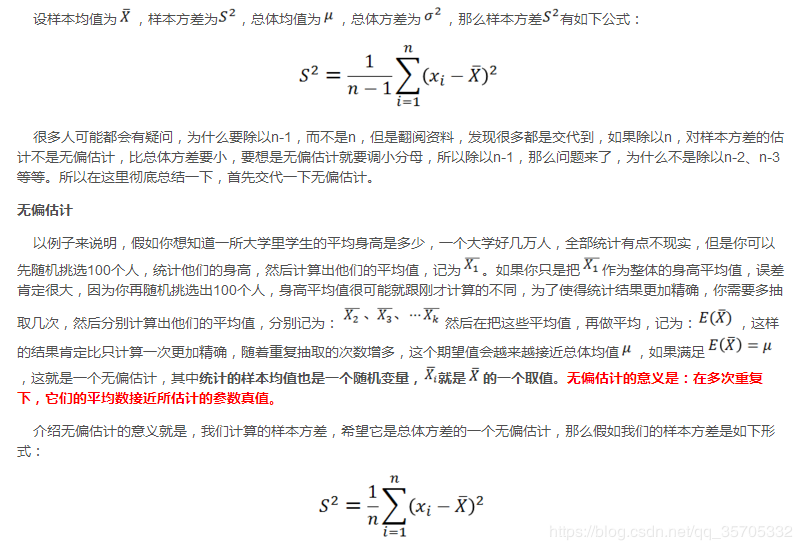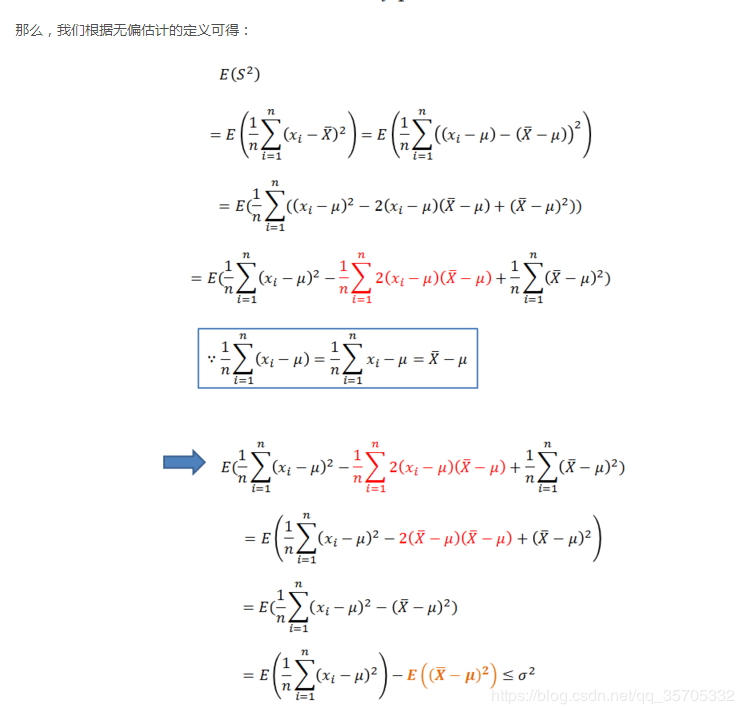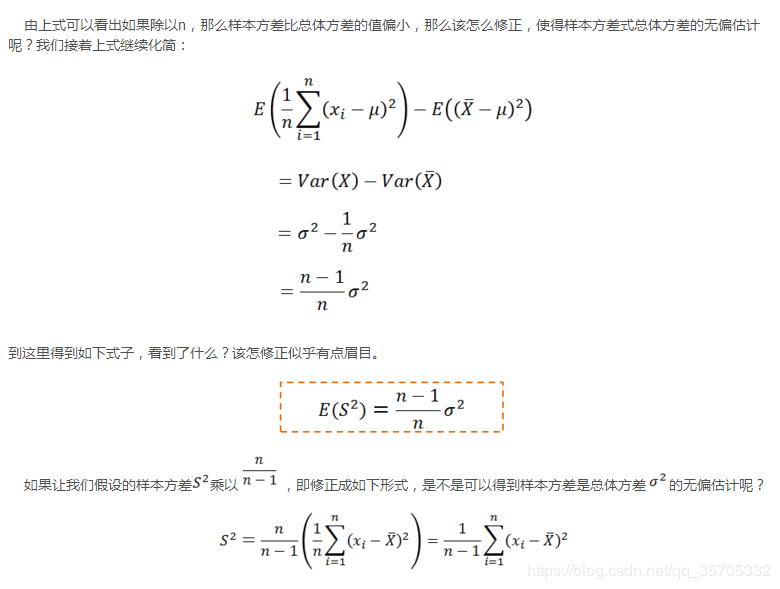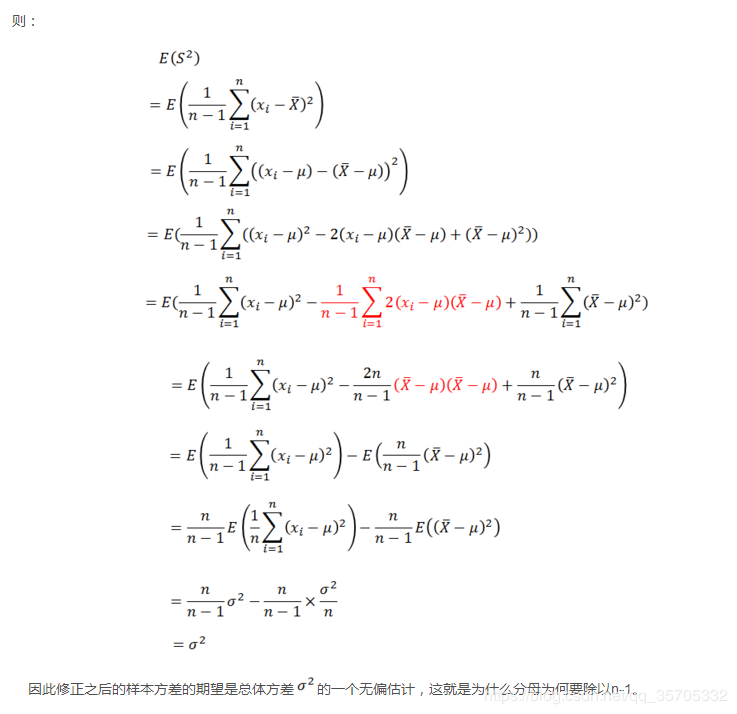展开全文贝叶斯理论
• 标准正态分布的概率密度公式正态分布概率密度公式多元正态分布的概率密度公式上式为 x 服从 k 元正态分布，x 为 k 维向量；|Σ| 代表协方差矩阵的行列式。二维正态分布概率密度函数为钟形曲面，等高线是椭圆线族，...
标准正态分布的概率密度公式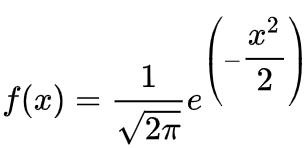正态分布概率密度公式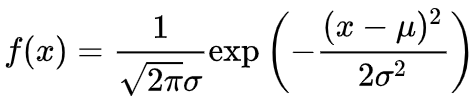多元正态分布的概率密度公式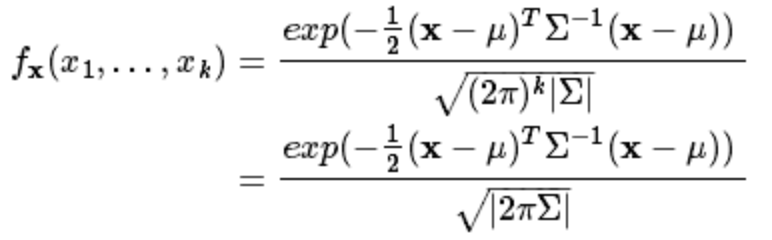上式为 x 服从 k 元正态分布，x 为 k 维向量；|Σ| 代表协方差矩阵的行列式。二维正态分布概率密度函数为钟形曲面，等高线是椭圆线族，并且二维正态分布的两个边缘分布都是一维正态分布，如图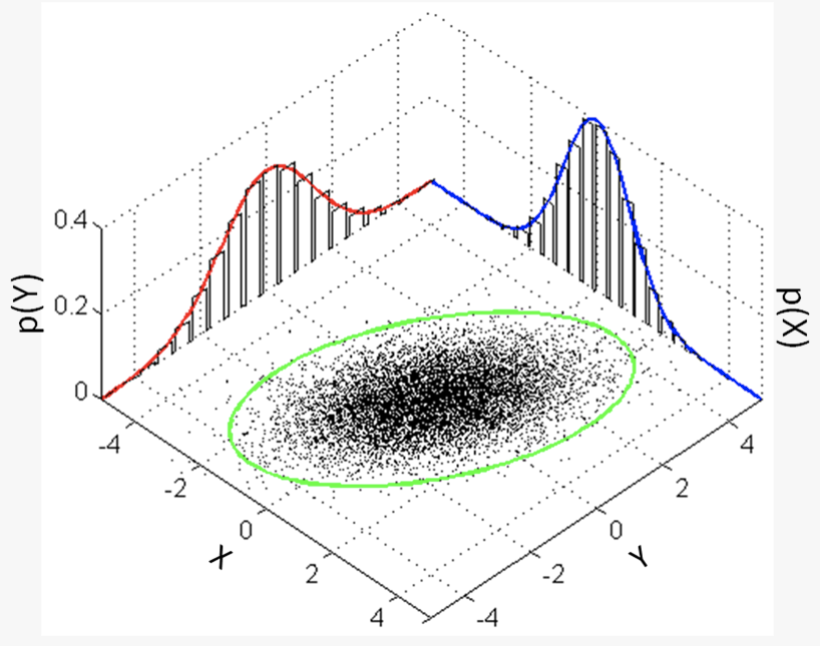numpy生成一个服从多元正态分布的数组multivariate_normal(mean, cov, size=None, check_valid=None, tol=None)各参数含义：mean：均值，维度为1，必选参数；cov：协方差矩阵，必选参数；size： 指定生成矩阵的维度，若size=(1, 1, 2)，则输出的矩阵的 shape 即形状为 1X1X2XN（N为mean的长度）；check_valid：可取值 warn，raise以及ignore；tol：检查协方差矩阵奇异值时的公差，float类型。示例：import numpy as npimport matplotlib.pyplot as pltmean = (1, 1)cov = np.array([[0.1, 0], [0, 1]])x = np.random.multivariate_normal(mean, cov, (500,), 'raise')   # nx2plt.scatter(x[:, 0], x[:, 1])plt.xlim(-3, 5)plt.ylim(-3, 5)plt.show()运行结果：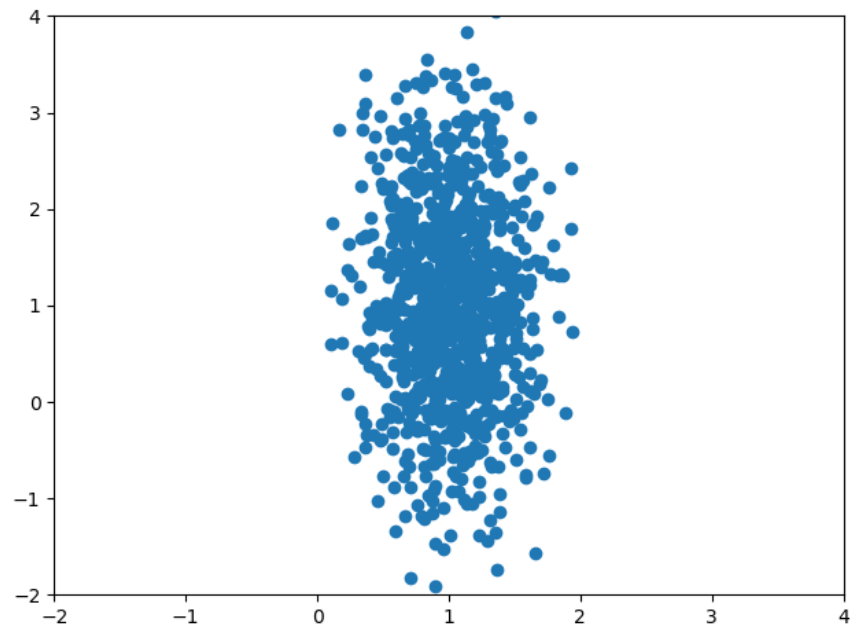参考资料
展开全文• 多元正态分布的检验多元正态分布mshapiro.test {mvnormtest}mvn {MVN} 多元正态分布 转自个人微信公众号【Memo_Cleon】的统计学习笔记：多元正态分布检验的R实现方法。 多元正态分布也称多元高斯分布。如同正态分布...数据分析 统计学 r语言
• 在本科阶段的教材中，往往会有多元正态分布的公式出现，但课堂上都不会重点讲解，而在研究生入学考试中也基本不会考。但在实际应用中，多元的情况却非常常见。 本文通过对多元正态分布的公式进行拆解，来看看它到底...统计学 统计模型 线性代数
• ## 多元正态分布

万次阅读 多人点赞 2014-06-03 09:32:15
多元正态分布 先定义一个d元随机向量，这里用列向量来表示，每一个元素都是一个一元随机变量，如  ,其转置为  其中表示这个多元随机变量的第i个分量，它是一个一维的随机变量。 高斯分布主要是用均值和方差来...
• 本文讨论了多元正态分布的定义，重点讨论多元正态分布的独立性、回归与最佳预测等问题。
• 此函数提供多元正态分布条件期望和协方差矩阵的矢量化估计。 均值是一个矩阵，其中行表示期望向量。 Sigma 是协方差矩阵。 Ind 是第一个无条件参数的索引。 值是条件值的矩阵，其中的行对应于平均行。matlab
• 多元正态分布的概率密度函数 N维随机向量 如果服从多变量正态分布，必须满足下面的三个等价条件： 任何线性组合 服 从正态分布。 存在随机向量 ( 它的每个元素服从独立标准正态分布），向量 及 矩阵A满足 存在 和一...
• 文章目录3.1 多元正态分布的定义二元正态分布例子 3.1 多元正态分布的定义 N(μ,σ2)N(\mu,\sigma^2)N(μ,σ2) f(x)=12πσe−(x−μ)22σ2f(x)=\frac{1}{\sqrt{2\pi}\sigma}e^{-\frac{(x-\mu)^2}{2\sigma^2}}f(x)...
• 1 ，多元正态分布 ： 数据 代码 ： x, y = np.random.multivariate_normal([0, 0], [[1, -.5], [-.5, 1]], size=300).T 2 ，核密度估计图 ：sns.kdeplot 含义 ： 根据已有数据，画图连线，推测数据走向 3 ，...python
• 算是一个学习指引吧，方便你找资料，其中也有部分底层代码，以及计算协方差的代码，代码都是经过验算的。涉及多元正态分布验算的部分，可以去寻找一元正态分布验算的代码，从而从曲线图像上观测样本拟合程度
• 假设X是有两个随机向量组成 其中 假设X服从多元高斯分布其中 多元正态分布
• 多元正态分布、聚类分析（系统、模糊、k均值、有序样品）
• 矩、协方差矩阵、多元正态分布的性质矩一元矩二元矩n元随机变量 X的数学期望(向量)n元随机变量 X~\widetilde{X}X的协方差矩阵n元正态随机变量的联合概率密度的矩阵表示n元正态随机变量的四条重要性质例1例2 ...
• 多元统计分析上机题之R语言实现（多元正态分布）-附件资源
• 多元正态分布 二维高斯型函数 http://comic.sjtu.edu.cn/thucs/GD_jsj_016b/text/chapter02/2_3_1t2.htm 1.多元正态分布的概率密度函数 　多元是指样本以多个变量来描述，或具有多个属性，在此一般用d维...
• X = rmvnrnd(MU,SIG,N,A,B) 在 N×P 矩阵 X a 中返回从 P 维多元正态中抽取的随机样本均值 MU 和协方差 SIG 截断为 a 的分布由不等式 Ax<=B 定义的超平面界定的区域。 [X,RHO,NAR,NGIBBS] = rmvnrnd(MU,SIG,N,A,B...matlab
• ## 多元正态分布的极大似然估计

万次阅读 多人点赞 2018-06-20 13:53:12
多元正态分布的极大似然估计 1. 一元正态分布的密度函数 一元正态分布的密度函数表示为： f(x)=1(2π)−−−−√σe−(x−μ)22σ2f(x)=1(2π)σe−(x−μ)22σ2f(x) = \frac{1}{\sqrt {(2 \pi)} \sigma} e^{...
• ## 多元正态分布的条件概率分布(一)

万次阅读 多人点赞 2014-06-04 12:25:20
多元正态分布的条件概率分布 假设分别有两个多维向量和 其中 那么的协方差矩阵为： 那么的协方差矩阵为： 那么的协方差矩阵为： 那么的协方差矩阵为...
• 在上两节理论知识的基础之上，我们在这一小节里以多元正态分布作为实际例子，让大家能够更直观的理解和强化这些概念和方法。 1.再谈相关性：基于多元正态分布 很简单，我们举一个例子，之前我们介绍过随机变量的正态...可视化 python 机器学习 人工智能 数据分析
• 简述多元正态分布下的最小错误率贝叶斯如果特征的值向量服从d元正态分布，即其概率密度函数为： 即其分布可以由均值向量和对称的协方差矩阵 唯一确定。如果认为样本的特征向量在类内服从多元正态分布： 即...机器学习 极大似然估计 最小错误率贝叶斯
• 多元正态分布具有两个参数——均值向量与自协方差函数，与数理统计一样，可以用抽样的方式定义一些统计量对它们进行参数估计。在这里，我们使用极大似然估计的方法，用样本均值和样本离差阵对它们进行估计。参数估计
• 这些知识，动不动就忘。为了不白选了一门数学课，还是把他...接下来附上3页草稿，验证二元正态分布的形式和多元正态分布的形式吻合: 如果n维正态分布的每一维相互独立，密度函数就是n个1维正态分布的乘积。
• 当一组变量服从多元正态分布时，如果根据样本的数据做出Scatterplot Matrix，那么每一幅Scatterplot上的散点都将聚集在一条直线附近。但要注意，从图像上来看，由于R的显示问题，以及方差的因素，看起来可能不那么像...线性代数
• 多元正态分布 1.1多元分布的基本概念 随机变量 假定所讨论的是多个变量的总体，所研究的数据是同时观测p 个指标（即变量），进行了n 次观测得到的，我们把这p 个指标表示为X1，X2，…，Xp，常用向量X ＝（X1，X2，...数据分析...